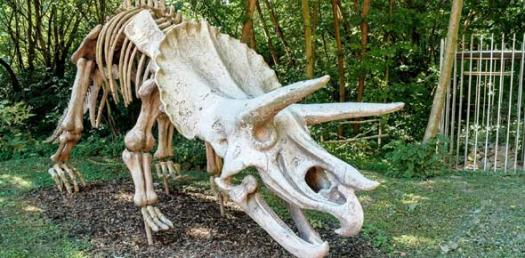# Fossils And Earth History

20 Questions | Total Attempts: 650SettingsThis quiz is testing your knowledge and understanding of types of fossils, dating methods and the three eras and time periods.

• 1.
The picture below can best be classified as what type of fossil?
• A.

Impression

• B.

Casts

• C.

Permineralization

• D.

Mold

• 2.
The following diagram best represents what type of fossil?
• A.

Permineralization

• B.

Impression

• C.

Amber

• D.

Gastrolith

• 3.
The diagram below can best be described as which type of fossil?
• A.

Carbonized imprint

• B.

Impression

• C.

Casts

• D.

Molds

• 4.
The picture below can best be described as which type of fossil?
• A.

Imprint

• B.

Impression

• C.

Casts

• D.

Permineralization

• E.

Freezing

• 5.
Scientists discovered this ancient beetle trapped in sand full of old crude oil based liquid. This can best be desribed as which type of fossil?
• A.

Carbon Imprint

• B.

Permineralization

• C.

Tar

• D.

Coprolite

• 6.
Which of the following would we use to measure the approximate age of Niagara Falls?
• A.

Rates of Erosion

• B.

Rates of Deposition

• C.

Varves

• D.

Carbon-14 Dating

• 7.
Which of the following listed layers would you expect to be the oldest?
• A.

Layer A

• B.

Layer B

• C.

Layer C

• D.

Layer D

• E.

Layer E

• 8.
If Layer C was the Mississipian Period, which of the following would make the most sense in classifying Layer B?
• A.

Paleozoic, Permian

• B.

Paleozoic, Cambrian

• C.

Mesozoic, Cambrian

• D.

Mesozoic, Triassic

• E.

Paleozoic, Tertiary

• 9.
If all of the layers were part of the Paleozoic Era, which of the following fossils would you NOT find in these rock layers?
• A.

Trilobites

• B.

Seed Bearing Plants

• C.

Primitive Sharks

• D.

Early Reptiles

• E.

Mammals

• 10.
Which of the following happened most recently?
• A.

Deposition of A

• B.

Magma intrusion of Layer E

• C.

Contact Metamorphism by Layer E

• D.

Earthquake between Layers B, C, D, and E

• 11.
If Layer A contained bones of deer and other mammals, which would be the best classification for Layer A?
• A.

Paleozoic, Permian

• B.

Mesozoic, Triassic

• C.

Mesozoic, Jurassic

• D.

Cenozoic, Tertiary

• 12.
Which fossil would best represent an index fossil?
• A.

Fossil 1

• B.

Fossil 2

• C.

Fossil 3

• 13.
The fossils in all three locations below are most likely from which era?
• A.

Cenozoic

• B.

Mesozoic

• C.

Paleozoic

• 14.
The following data table shows the amount of radioactive Radium decreasing over time. Examine the data and decide what the half life is for this radioactive element. Amount (# of atoms of Ra-222) Time (Minutes)  24, 000 0 min 22,000 8 min 20,000 16 min 18,000 24 min 16,000 32 min 14,000 40 min 12,000 48 min 10,000 64 min 8,000 80 min 6,000 96 min
• A.

8 min

• B.

24 min

• C.

48 min

• D.

96 min

• 15.
If one started with 400 atoms of plutonium-239 and now only have 12.5 grams,             a. how many half-lives occurred?
• A.

2

• B.

3

• C.

4

• D.

5

• E.

6

• 16.
If a fossil contained 75% of the daughter element and 25% of the original radioactive parent element, how many half lives occurred?
• A.

0

• B.

1

• C.

2

• D.

3

• E.

4

• 17.
If radioactive Polonium, Po-197, has a half life of four minutes, how much Po-197 will remain after 24 minutes if we originally started with 200 grams?
• A.

50 grams

• B.

25 grams

• C.

• D.

• E.

• 18.
Which of the following rock layers would be the youngest?
• A.

1

• B.

6

• C.

4

• D.

3

• 19.
Which of the following would be the OLDEST rock layer?
• A.

3

• B.

2

• C.

5

• D.

4

• 20.
Is there evidence that an earthquake occurred?
• A.

YES, along A

• B.

NO

• C.

YES, along B

Related Topics